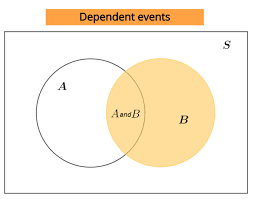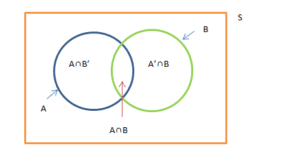## How to Calculate and Solve for Dependent Events | ProbabilityThe image above represents dependent events.

To compute for dependent events, four essential parameters are needed and these parameters are Number of Times Event A can occur (xA), Number of Times Event B can occur (xB) and Total Number of All Possible Outcomes (N).

The formula for calculating independent events:

P(A and B) = P(A) x P(B|A)

Where;

P(A and B) = Dependent events
xA = Number of Times Event A can occur
xB = Number of Times Event B can occur
N = Total Number of All Possible Outcomes
P(A) = xAN
P(B|A) = xB(N – 1)

Let’s solve an example;
Find the dependent events when the number of times event A can occur is 8, number of times event B can occur is 11 and the total number of all possible outcomes is 18.

This implies that;

xA = Number of Times Event A can occur = 8
xB = Number of Times Event B can occur = 11
N = Total Number of All Possible Outcomes = 18

P(A and B) = P(A) x P(B|A)
P(A and B) = xAN x xB(N – 1)
P(A and B) = 818 x 1117
P(A and B) = (8)(11)(18)(17)
P(A and B) = 88306
Dividing the numerator and denominator by 2
P(A and B) = 44153
P(A and B) = 0.287

Therefore, the dependent events is 0.287.

## How to Calculate and Solve for Independent Events | ProbabilityThe image above represents independent events.

To compute for independent events, four essential parameters are needed and these parameters are Number of Times Event A can occur (xA), Number of Times Event B can occur (xB) and Total Number of All Possible Outcomes (N).

The formula for calculating independent events:

P(A and B) = P(A) x P(B)

Where;

P(A and B) = Independent events
xA = Number of Times Event A can occur
xB = Number of Times Event B can occur
N = Total Number of All Possible Outcomes
P(A) = xAN
P(B) = xBN

Let’s solve an example;
Find the independent events when the number of times event A can occur is 11, number of times event B can occur is 15 and the total number of all possible outcomes is 22.

This implies that;

xA = Number of Times Event A can occur = 11
xB = Number of Times Event B can occur = 15
N = Total Number of All Possible Outcomes = 22

P(A and B) = P(A) x P(B)
P(A and B) = xAN x xBN
P(A and B) = 1122 x 1522
P(A and B) = (11)(15)(22)(22)
P(A and B) = 165484
Dividing the numerator and denominator by 11
P(A and B) = 1544
P(A and B) = 0.3409

Therefore, the independent events is 0.3409.